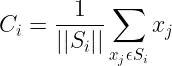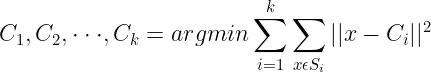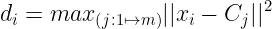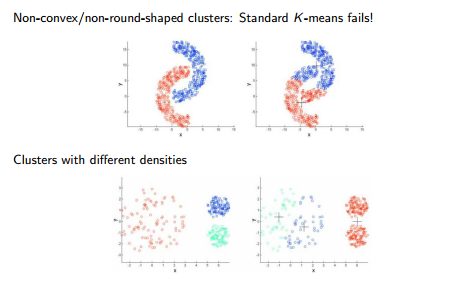This article was published as a part of the Data Science Blogathon

## Introduction

Clustering Algorithms come in handy to use when the dataset provided in the problem statement is not labelled and therefore can not be predicted using supervised learning techniques.

Among the unsupervised techniques used K means algorithm is the most important algorithm that helps to cluster the data on the basis of their similarity.

Therefore it becomes necessary for every aspiring Data Scientist and Machine Learning Engineer to have a good knowledge of these techniques.

In this article, we will discuss the most important questions on K means Clustering Algorithm which is helpful to get you a clear understanding of the algorithm, and also for Data Science Interviews, which covers its very fundamental level to complex concepts.

Let’s get started!

## 1. What is K means Clustering Algorithm?

K Means algorithm is a centroid-based clustering (unsupervised) technique. This technique groups the dataset into k different clusters having an almost equal number of points. Each of the clusters has a centroid point which represents the mean of the data points lying in that cluster.The idea of the K-Means algorithm is to find k-centroid points and every point in the dataset will belong to either of the k-sets having minimum Euclidean distance.

## 2. What is Lloyd’s algorithm for Clustering?

It is an approximation iterative algorithm that is used to cluster the data points.The steps of this algorithm are as follows:

• Initialization
• Assignment
• Update Centroid
• Repeat Steps 2 and 3 until convergence

### Step-1: Initialization

Randomly initialized k-centroids from the data points.

### Step-2: Assignment

For each observation in the dataset, calculate the euclidean distance between the point and all centroids. Then, assign a particular observation to the cluster with the nearest centroid.

### Step-3: Updation of Centroid

Now, observations in the clusters are changed. Therefore, update the value of the centroid with the new mean(average of all observations)value.

### Step-4: Repeat Steps 2 and 3 until convergence

Repeat steps 2 and 3 until the algorithm converges. If convergence is achieved then break the loop. Convergence refers to the condition where the previous value of centroids is equal to the updated value after the algorithm run.

## 3. Is Feature Scaling required for the K means Algorithm?

Yes, K-Means typically needs to have some form of normalization done on the datasets to work properly since it is sensitive to both the mean and variance of the datasets.For performing feature scaling, generally, StandardScaler is recommended, but depending on the specific use cases, other techniques might be more suitable as well.

For Example, let’s have 2 variables, named age and salary where age is in the range of 20 to 60 and salary is in the range of 100-150K, since scales of these variables are different so when these variables are substituted in the euclidean distance formula, then the variable which is on the large scale suppresses the variable which is on the smaller scale. So, the impact of age will not be captured very clearly. Hence, you have to scale the variables to the same range using Standard Scaler, Min-Max Scaler, etc.

## 4. Why do you prefer Euclidean distance over Manhattan distance in the K means Algorithm?

Euclidean distance is preferred over Manhattan distance since Manhattan distance calculates distance only vertically or horizontally due to which it has dimension restrictions.On the contrary, Euclidean distance can be used in any space to calculate the distances between the data points. Since in K means algorithm the data points can be present in any dimension, so Euclidean distance is a more suitable option.

## 5. Why is the plot of the within-cluster sum of squares error (inertia) vs K in K means clustering algorithm elbow-shaped? Discuss if there exists any other possibility for the same with proper explanation.

Let’s understand this with an example,Say, we have 10 different data points present, now consider the different cases:

• k=10: For the max value of k, all points behave as one cluster. So, within the cluster sum of squares is zero since only one data point is present in each of the clusters. So, at the max value of k, this should tend to zero.
• K=1: For the minimum value of k i.e, k=1, all these data points are present in the one cluster, and due to more points in the same cluster gives more variance i.e, more within-cluster sum of squares.
• Between K=1 from K=10: When you increase the value of k from 1 to 10, more points will go to other clusters, and hence the total within the cluster sum of squares (inertia) will come down. So, mostly this forms an elbow curve instead of other complex curves.

Hence, we can conclude that there does not exist any other possibility for the plot.

## 6. Which metrics can you use to find the accuracy of the K means Algorithm?

There does not exist a correct answer to this question as k means being an unsupervised learning technique does not discuss anything about the output column. As a result, one can not get the accuracy number or values from the algorithm directly.

## 7. What is a centroid point in K means Clustering?

Centroid point is the point that acts as a representative of a particular cluster and is the average of all the data points in the cluster which changes in each step (until convergence). Centroid can be calculated using the given formula:where,

Ci: ith Centroid

Si: All points belonging to set-i with centroid as Ci

xj: jth point from the set

||Si||: number of points in set-i

## 8. Does centroid initialization affect K means Algorithm?

Yes, the final results of the k means algorithm depend on the centroid initialization as poor initialization can cause the algorithm to get stuck into an inferior local minimum.

## 9. Discuss the optimization function for the K means Algorithm.The objective of the K-Means algorithm is to find the k (k=no of clusters) number of centroids from C1, C2,——, Ck which minimizes the within-cluster sum of squares i.e, the total sum over each cluster of the sum of the square of the distance between the point and its centroid.

This cost comes under the NP-hard problem and therefore has exponential time complexity. So we come up with the idea of approximation using Lloyd’s Algorithm.

## 10. What are the advantages and disadvantages of the K means Algorithm?

• Easy to understand and implement.
• Computationally efficient for both training and prediction.
• Guaranteed convergence.

• We need to provide the number of clusters as an input variable to the algorithm.
• It is very sensitive to the initialization process.
• Good at clustering when we are dealing with spherical cluster shapes, but it will perform poorly when dealing with more complicated shapes.
• Due to the leveraging of the euclidean distance function, it is sensitive to outliers.

## 11. What are the challenges associated with K means Clustering?

The major challenge associated with k means clustering is its initialization sensitivity.While finding the initial centroids for K-Means clustering using Lloyd’s algorithm, we were using randomization i.e, initial k-centroids were picked randomly from the data points.

This Randomization in picking the k-centroids creates the problem of initialization sensitivity which tends to affect the final formed clusters. As a result, the final formed clusters depend on how initial centroids were picked.

## 12. What are the ways to avoid the problem of initialization sensitivity in the K means Algorithm?

There are two ways to avoid the problem of initialization sensitivity:

• Repeat K means: It basically repeats the algorithm again and again along with initializing the centroids followed by picking up the cluster which results in the small intracluster distance and large intercluster distance.
• K Means++: It is a smart centroid initialization technique.

Amongst the above two techniques, K-Means++ is the best approach.

## 13. What is the difference between K means and K means++ Clustering?

In k-means, we randomly initialized the k number of centroids while in the k-means++ algorithm, firstly we initialized 1 centroid and for other centroids, we have to ensure that the next centroids are very far from the initial centroids which result in a lower possibility of the centroid being poorly initialized. As a result, the convergence is faster in K means++ clustering.Moreover, in order to implement the k-means++ clustering using the Scikit-learn library, we set the parameters to init = kmeans++ instead of random.

## 14. How K means++ clustering Algorithm works?

K Means++ algorithm is a smart technique for centroid initialization that initialized one centroid while ensuring the others to be far away from the chosen one resulting in faster convergence.The steps to follow for centroid initialization are:

Step-1: Pick the first centroid point randomly.

Step-2: Compute the distance of all points in the dataset from the selected centroid. The distance of xi point from the farthest centroid can be calculated by the given formula:where,

di: Distance of xi point from the farthest centroid

m: number of centroids already picked

Step-3: Make the point xi as the new centroid that is having maximum probability proportional to di

Step-4: Repeat the above last two steps till you find k centroids.

## 15. How to decide the optimal number of K in the K means Algorithm?

Most of the people give answers to this question directly as the Elbow Method however the explanation is only partially correct.In order to find the optimal value of k, we need to observe our business problem carefully, along with analyzing the business inputs as well as the person who works on that data so that a decent idea regarding the optimal number of clusters can be extracted.

For Example, If we consider the data of a shopkeeper selling a product in which he will observe that some people buy things in summer, some in winter while some in between these two. So, the shopkeeper divides the customers into three categories. Therefore, K=3.

In cases where we do not get inference from the data directly we often use the following mentioned techniques:

• Elbow Method – This method finds the point of inflection on a graph of the percentage of variance explained to the number of K and finds the elbow point.
• Silhouette method – The silhouette method calculates similarity/dissimilarity score between their assigned cluster and the next best (i.e, nearest) cluster for each of the data points.

Moreover, there are also other techniques along with the above-mentioned ones to find the optimal no of k.

## 16. What is the training and testing complexity of the K means Algorithm?

Training complexity in terms of Big-O notation:If we use Lloyd’s algorithm, the complexity for training is: “K*I*N*M”

where,

K: It represents the number of clusters

I: It represents the number of iterations

N: It represents the sample size

M: It represents the number of variables

Conclusion: There is a significant Impact on capping the number of iterations.

Predicting complexity in terms of Big-O notation:

“K*N*M”

Prediction needs to be computed for each record, the distance to each cluster and assigned to the nearest ones.

## 17. Is it possible that the assignment of data points to clusters does not change between successive iterations in the K means Algorithm?

When the K-Means algorithm has reached the local or global minima, it will not change the assignment of data points to clusters for two successive iterations during the algorithm run.

## 18. Explain some cases where K means clustering fails to give good results.

The K means clustering algorithm fails to give good results in the below-mentioned cases:

• When the dataset contains outliers
• When the density spread of data points across the data space is different.
• When the data points follow a non-convex shape.## 19. How to perform K means on larger datasets to make it faster?

The idea behind this is mini-batch k means, which is an alternative to the traditional k means clustering algorithm that provides better performance for training on larger datasets.It leverages the mini-batches of data, taken at random to update the cluster mean with a decreasing learning rate. For each data batch, the points are all first assigned to a cluster and then means are re-calculated. The cluster centres are then further re-calculated using gradient descent. This algorithm provides faster convergence than the typical k-means, but with a slightly different cluster output.

## 20. What are the possible stopping conditions in the K means Algorithm?

The following can be used as possible stopping conditions in K-Means clustering:

• Max number of iterations has been reached: This condition limits the runtime of the clustering algorithm, but in some cases, the quality of the clustering will be poor because of an insufficient number of iterations.
• When RSS(within-cluster sum of squares) falls below a threshold: This criterion ensures that the clustering is of the desired quality after termination. Practically in real-life problems, it’s a good practice to combine it with a bound on the number of iterations to guarantee convergence.
• Convergence: Points stay in the same cluster i.e., the algorithm has converged at the minima.
• Stability: Centroids of new clusters do not change.

## 21. What is the effect of the number of variables on the K means Algorithm?

The number of variables going into K means the algorithm has an impact on both the time(during training) and complexity(upon application) along with the behaviour of the algorithm as well.This is also related to the “Curse of dimensionality”. As the dimensionality of the dataset increases, more and more examples become nearest neighbours of xt, until the choice of nearest neighbour is effectively random.

A key component of K means is that the distance-based computations are directly impacted by a large number of dimensions since the distances between a data point and its nearest and farthest neighbours can become equidistant in high dimension thereby resulting in reduced accuracy of distance-based analysis tools.

Therefore, we have to use the Dimensionality reduction techniques such as Principal component analysis (PCA), or Feature Selection Techniques.

## End Notes

I hope you enjoyed the questions and were able to test your knowledge about K means Clustering Algorithm.

If you liked this and want to know more, go visit my other articles on Data Science and Machine Learning by clicking on the Link

Something not mentioned or want to share your thoughts? Feel free to comment below And I’ll get back to you.

## Chirag Goyal

Currently, I pursuing my Bachelor of Technology (B.Tech) in Computer Science and Engineering from the Indian Institute of Technology Jodhpur(IITJ). I am very enthusiastic about Machine learning, Deep Learning, and Artificial Intelligence.

The media shown in this article are not owned by Analytics Vidhya and is used at the Author’s discretion.